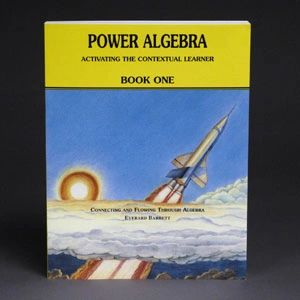Free Shipping on all U.S. Orders!# Power Algebra Book 1

Professor B

• \$85.00
Unit price per
Shipping calculated at checkout.

## Algebra Book One - Physical Product

Professor B methodology carefully nurtures children's perception of connection and flow in arithmetic so they experience enjoyment and mastery of math content. To nurture learners' perception of connection and flow, Professor B Algebra integrates Pre-algebra, Algebra I and Algebra II into a single, ungraded curriculum in three volumes.  Each concept is covered thoroughly, with clarity.

Each textbook contains the answers and can usually be completed in 2 - 3 years, even by children as young as 9 who have completed Prof. B Level 3.

Algebra Book One is a physical product (8.5 x 11 inches paperback) and can be used as a text only with the student writing their answers in a notebook, or can be used as a consumable workbook, as the exercises are included.   Algebra Book 2 and Algebra Book 3 are attached as Digital Downloads to your email at the time of purchase.

• Introducing Integers
• Adding Integers and Three Methods for Subtracting Integers
• Simplifying Expressions Involving Sums and Differences of Integers and Combining Like Terms
• Multiplying and Dividing with Integers
• Multiplying and Dividing with Powers The Positive, Negative and Zero Exponents
• Multiplication/Division with Monomials and Expressions Involving Negative and Zero Exponents
• Evaluation of Algebraic Expressions and Order of Operations
• Adding and Subtracting with Polynomials
• Multiplying with Polynomials and Factoring by Removing the Highest Common Factor
• Multiplying with Polynomials and Factoring Trinomials of the Form ax² + bx + c and ax² + bxy + cy²
• Multiplying with Polynomials and Factoring the Difference of Two Squares
• Multiplying with Polynomials and Factoring the Sum and Difference of Two Cubes
• Multiplying with Polynomials and Factoring by Grouping Multiplying with Polynomials and More Advanced Factoring Multiplying with Polynomials and Factoring
• Completely Dividing with Polynomials and Synthetic Division
• Binomial Expansion
• Distinguishing Between Sums and Products – The Real Numbers –
• Reducing Rational Expressions to Lowest Terms
• Multiplying and Dividing with Rational Expressions